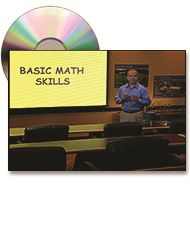•# AWWA-64127 Operator Math Made Easy DVD

\$169.00
Video Preview
Operators learn many commonly used water treatment calculations.

Operator Math Made Easy DVD teaches water operators mathematical calculations used in water treatment and distribution. It is an ideal training tool for novice water treatment operators and an excellent reference on the job.

Topics covered:
• Powers notation
• Scientific notation
• Dimensional analysis
• Rounding and estimating
• Solving for the unknown value
• Ratio and proportion
• Average
• Percentage
• Circumference
• Surface area
• Volume
• Conversion tables
• Graphs
• Average Daily Flow
• Surface Overflow Rate
• Weir Overflow Rate